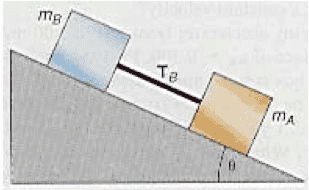# Two Blocks attached by a string, on an incline

## Homework Statement

In the diagram mA is equal to 3.00 kg and mB is equal to 1.07 kg. The angle of the inclined plane is 38.0°(a) Find Acceleration: 6.03 m/s^2 (got this part)
(b) Find the tension Tb in the connecting string: Not sure

## Homework Equations

(a) a = (F/mA + mB)
(b) t = mA * a? or mB * a? Not sure

## The Attempt at a Solution

Obviously my mA * a and mB * a both didn't work, I'm not sure what the formula should be.

Doc Al
Mentor
What forces act on each block? Draw yourself a free body diagram for one of the masses. Then by applying Newton's 2nd law, you can solve for the tension.

I'm sorry, but... What?

The force that acts on each block is gravity, 9.8 * sin theta. That's how I got acceleration.

T = m(g+a)? But is that mA or mB?

Doc Al
Mentor
I'm sorry, but... What?

The force that acts on each block is gravity, 9.8 * sin theta. That's how I got acceleration.
Right, but you left it out in your two formulas for tension.

T = m(g+a)? But is that mA or mB?
Do this. Pick a block. Doesn't matter which. Then find ∑F for that block. Direction matters: call down the incline positive.

•1 person
Right, but you left it out in your two formulas for tension.

Woops, sorry. O_O I missed that.

Do this. Pick a block. Doesn't matter which. Then find ∑F for that block. Direction matters: call down the incline positive.

3 * (9.8 + 6.03) = 47.49

I don't know what the summation F means. Sum of forces on the block?

I usually have practice problems so I can play with them until I figure out how to do them, no such luck on this one.

Doc Al
Mentor
I don't know what the summation F means. Sum of forces on the block?
Yes.

Did you pick a block? Which one? What forces act on it? What's their sum?